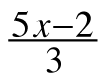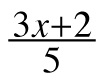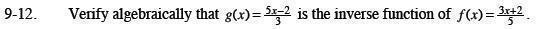Home > CCA2 > Chapter 9 > Lesson 9.1.1 > Problem9-12

9-12.

Verify algebraically that g(x) =is the inverse function of f(x) =. Homework Help ✎Substitute f(x) into g(x). Simplify.

$g(f(x))=\frac{5\left(\frac{3x+2}{5}\right)-2}{3}=x$

Substitute g(x) into f(x). Simplify.

$f(g(x))=\frac{3\left(\frac{5x-2}{3}\right)+2}{5}=x$

Since f(g(x)) = g(f(x)) = x, they are inverse functions.